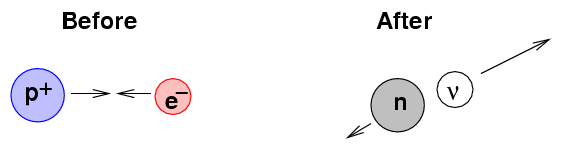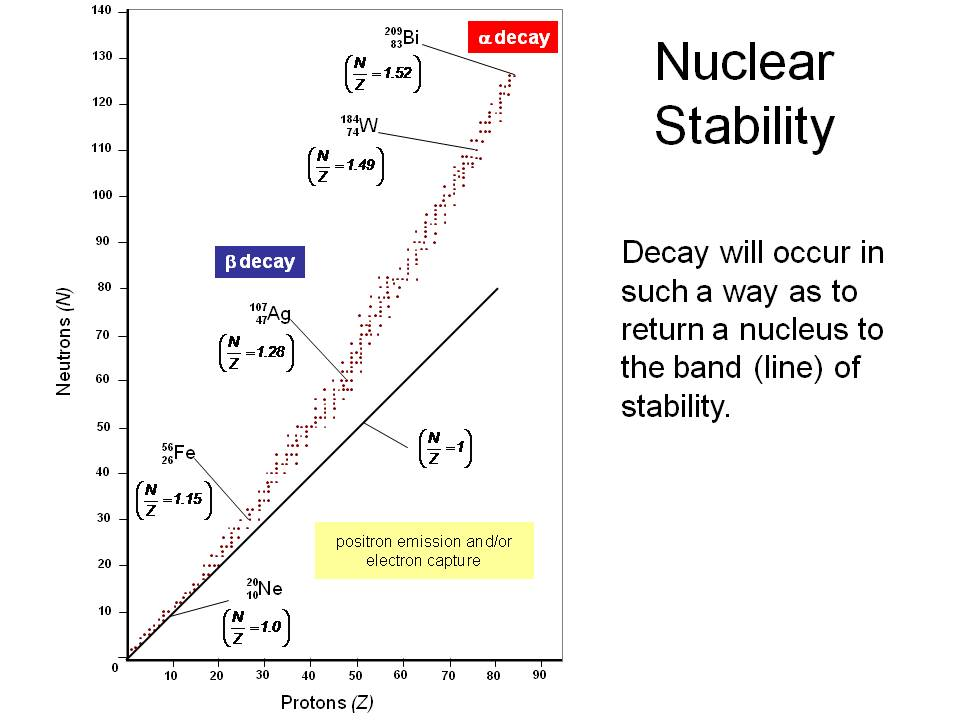# How does electron capture affect the neutron-proton ratio?

Oct 7, 2014

Electron capture increases the neutron-proton ratio.

Electron capture is a process in which a nucleus with too many protons absorbs an electron from an inner orbital.

This changes a proton to a neutron and simultaneously emits an electron neutrino.For example.

""_-1^0"e" + _4^7"Be" → _3^7"Li" + ν_e

The $\text{_4^7"Be}$ has 4 protons and 3 neutrons.

After electron capture, the new $\text{_3^7"Li}$ has 3 protons and 4 neutrons. The $n : p$ ratio is 4:3 = 1.33:1.

The $n : p$ ratio has increased from 0.75:1 to 1.33:1. So electron capture has increased the n:p ratio.

A nucleus with too many protons lies below the band of stability.It will undergo either electron capture or positron emission to reduce the number of protons (and increase the number of neutrons) to get back to the band of stability.